Harmonic Mean - Measures of Central Tendency, Business Mathematics & Statistics

# Harmonic Mean - Measures of Central Tendency, Business Mathematics & Statistics - Business Mathematics and Statistics - B Com

HARMONIC MEAN (H. M.) :

Definition. The Harmonic Mean (H) for n observations, x1 , x2 ,…….xn is the total number divided by the sum of the reciprocals * of the numbers.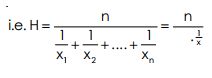Again,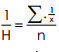(i.e. reciprocal of H. M = A. M. of reciprocals of the numbers).

* For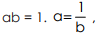, i.e. a reciprocal of b. And for 1/ a = b, b is reciprocal of a. Reciprocal of 2 is. 1/2 .

Example 17 : Find the H. M. of 3, 6, 12 and 15.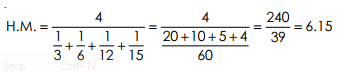Example 18 : Find the H.M. of 1, 1/2 , 1/3 ,……. 1/n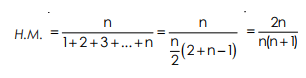[Note. The denominator is in A..P. use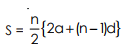Example 19 : A motor car covered distance of 50 miles four times. The first time at 50 m. p. h, the second at 20 m. p. h., the third at 40 m. p. h, and the fourth at 25 m.p.h Calculate the average speed and explain the choice of the average.

Average Speed (H.M) =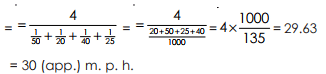For the statement x units per hour, when the different values of x (i.e. distances) are given, to find average, use H.M. If again hours (i.e., time of journey) are given, to find average, we are to use A.M. In the above example, miles (distances) are given, so we have used H.M. Weighted H.M. The formula to be used is as follows :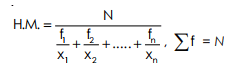Example 20 : (a) A person travelled 20 k.m. at 5 k.m.p.h. and again 24 k.m. at 4 k.m.p.; to find average speed. (b) A person travelled 20 hours at 5 k.m.p.h. and again 24 hours at 4.m.p.h.; to find average speed. (a) We are to apply H.M. (weight) in this case, since, distances are given.

Average speed (H.M.)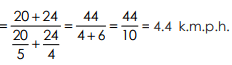Average speed (A.M.)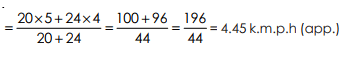Example 21 : Find the harmonic mean of the following numbers :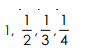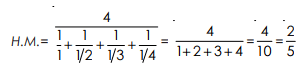Example 22 : An aeroplane flies around a square and sides of which measure 100 kms. Each. The aeroplane cover at a speed of 10 Kms per hour the first side, at200 kms per hour the second side, at 300 kms per hour the third side and at 400 kms per hour the fourth side. Use the correct mean to find the average speed round the square.
Here H.M. is the appropriate mean.
Let the required average speed be H kms per hours

then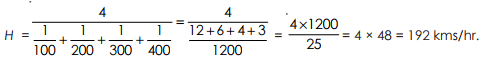(i) Like A.M. and G. M. it is also based on all observations.
(ii) Capable of further algebraic treatment.
(iii) It is extremely useful while averaging certain types of rates and rations.

(i) It is not readily understood nor can it be calculated with ease.
(ii) It is usually a value which may not be a member of the given set of numbers.
(iii) It cannot be calculated when there are both negative and positive values in a series or one of more values in zero.

It is useful in averaging speed, if the distance travelled is equal. When it is used to give target weight to smallest item, this average is used.

The document Harmonic Mean - Measures of Central Tendency, Business Mathematics & Statistics | Business Mathematics and Statistics - B Com is a part of the B Com Course Business Mathematics and Statistics.
All you need of B Com at this link: B Com

115 videos|142 docs

## FAQs on Harmonic Mean - Measures of Central Tendency, Business Mathematics & Statistics - Business Mathematics and Statistics - B Com

 1. What is the harmonic mean and how is it calculated?Ans. The harmonic mean is a measure of central tendency used in statistics. It is calculated by taking the reciprocal of the arithmetic mean of the reciprocals of a set of values. In other words, it is the reciprocal of the average of the reciprocals. The formula for calculating the harmonic mean is: Harmonic Mean = n / (1/x1 + 1/x2 + ... + 1/xn) where n is the number of values and x1, x2, ..., xn are the individual values.
 2. When is the harmonic mean used instead of the arithmetic mean?Ans. The harmonic mean is often used in situations where rates or ratios are involved. It is particularly useful when dealing with average rates of speed, such as calculating average speed in a round trip. The harmonic mean gives more weight to smaller values, making it a better representation of the overall rate.
 3. Can the harmonic mean be greater than the arithmetic mean?Ans. No, the harmonic mean is always smaller than the arithmetic mean. This is because the harmonic mean gives more weight to smaller values. As a result, the harmonic mean tends to be pulled towards the smaller values in the set, making it smaller overall.
 4. What are the advantages of using the harmonic mean?Ans. The harmonic mean has several advantages in certain situations. It is less affected by extreme values or outliers in the data compared to the arithmetic mean. It is also a useful measure when dealing with rates or ratios, as mentioned earlier. Additionally, the harmonic mean ensures that the average value is not skewed by extremely large or small values in the data set.
 5. Can the harmonic mean be calculated for negative values?Ans. No, the harmonic mean cannot be calculated for negative values. The reciprocal of a negative number is not defined, so the formula for the harmonic mean does not work for negative values. The data set used to calculate the harmonic mean should only consist of positive values.

115 videos|142 docsExplore Courses for B Com examSignup to see your scores go up within 7 days! Learn & Practice with 1000+ FREE Notes, Videos & Tests.
10M+ students study on EduRev
Track your progress, build streaks, highlight & save important lessons and more!
Related Searches

,

,

,

,

,

,

,

,

,

,

,

,

,

,

,

,

,

,

,

,

,

,

,

,

;